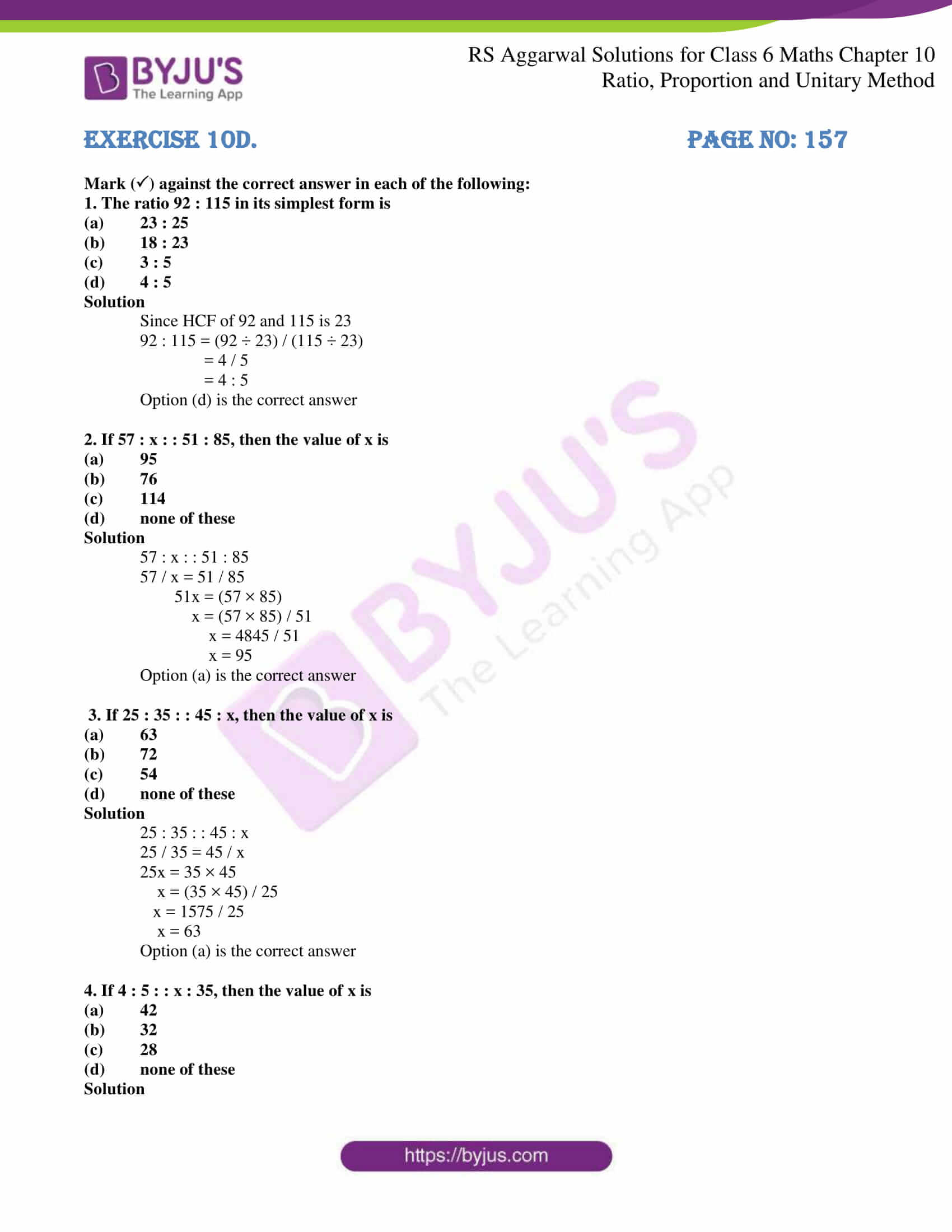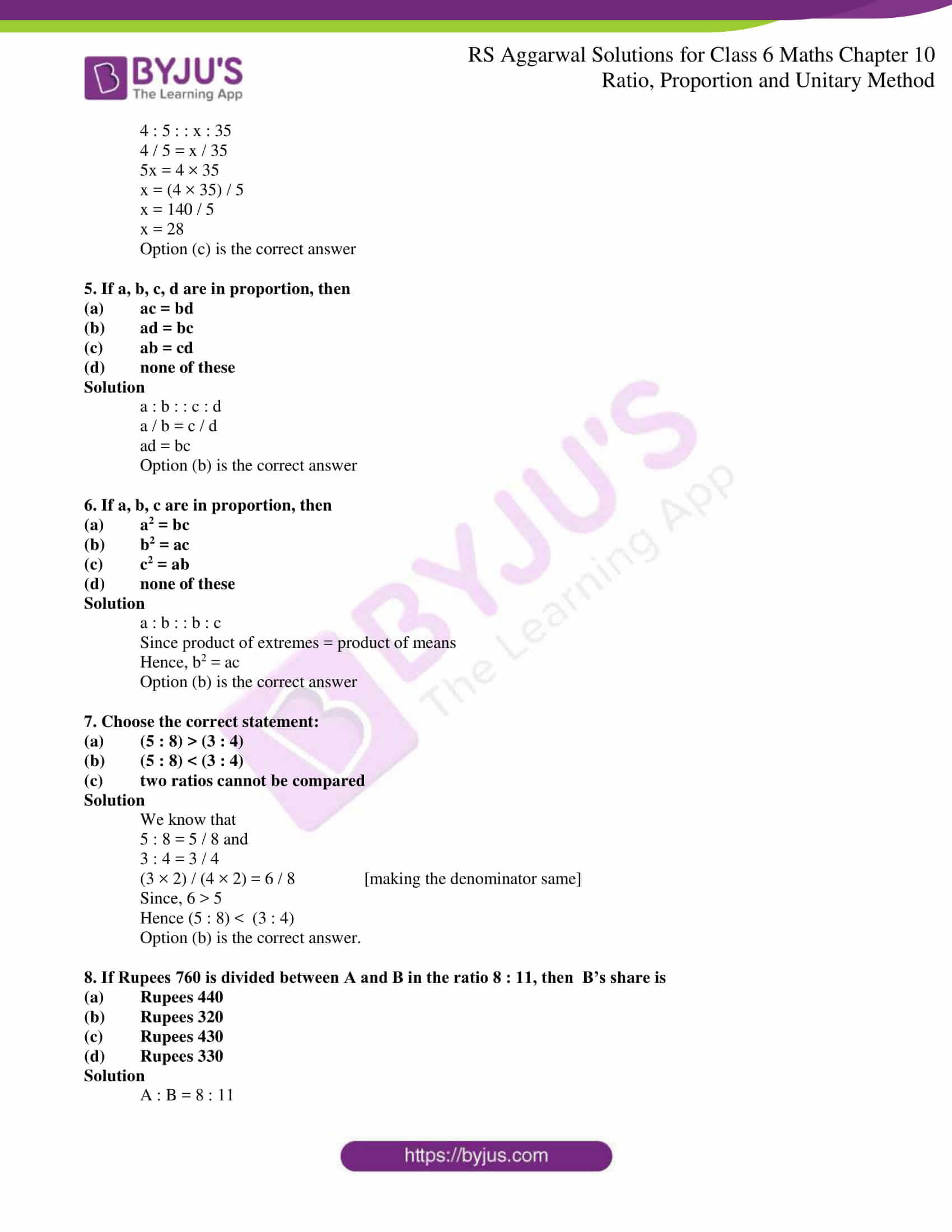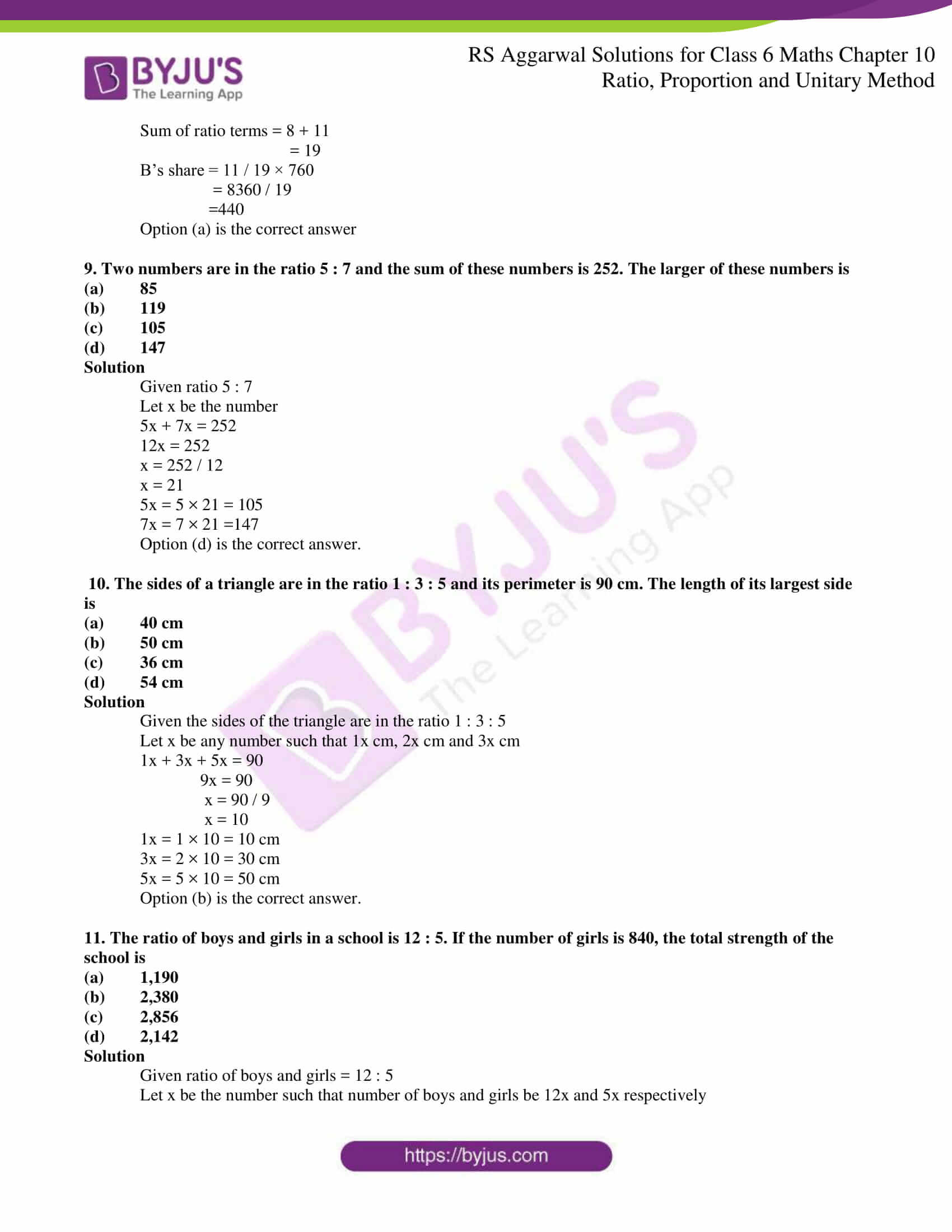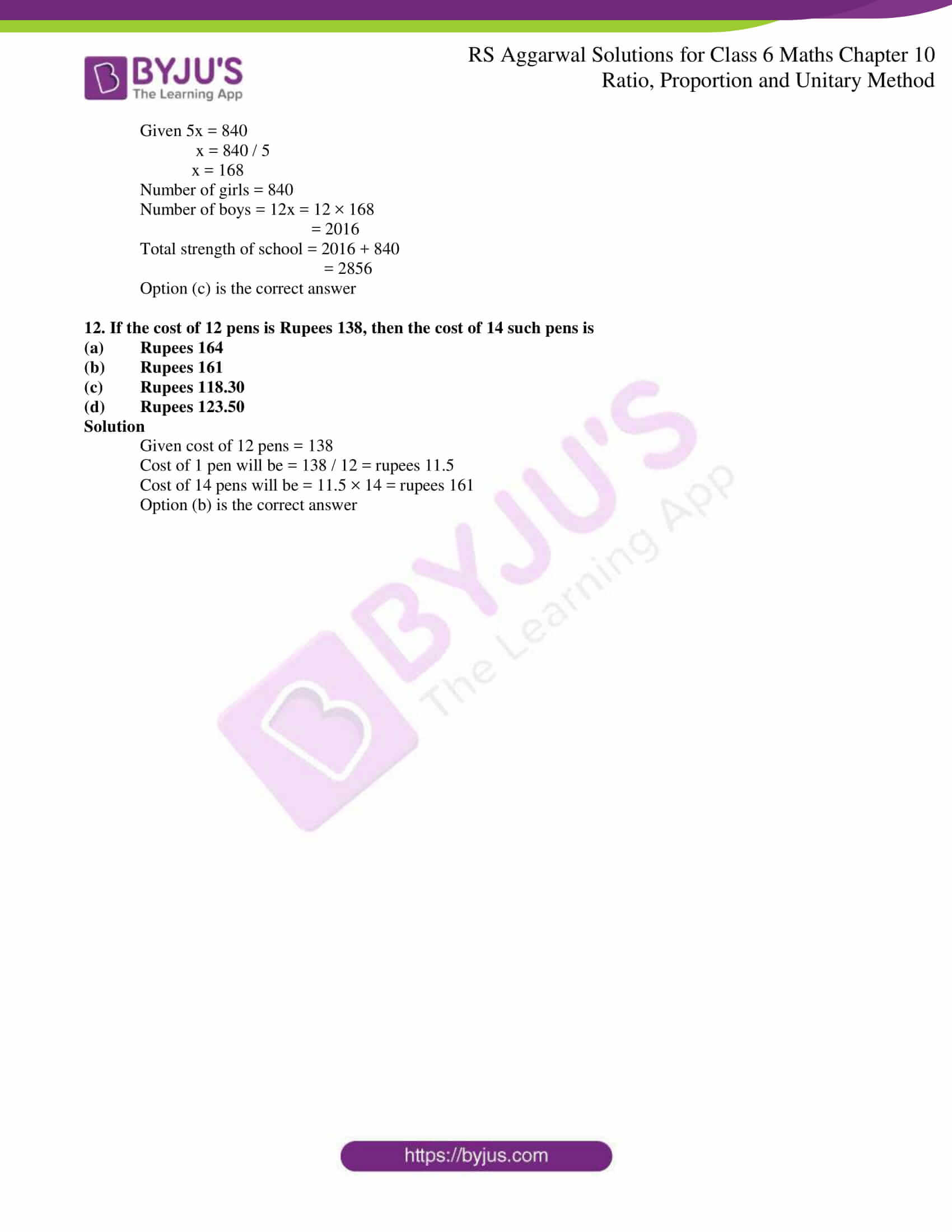# RS Aggarwal Solutions for Class 6 Chapter 10 Ratio, Proportion and Unitary Method Exercise 10D

The objective type problems in this chapter mainly help students in preparing themselves for Class 6 exams and other competitive exams. RS Aggarwal Solutions consists of these problems which are solved using various shortcut tips and formulas to help students grasp the concepts faster. The students can access the RS Aggarwal Solutions for Class 6 Chapter 10 Ratio, Proportion and Unitary Method Exercise 10C PDF to get a better knowledge about the methods of solving problems.

## Download PDF of RS Aggarwal Solutions for Class 6 Chapter 10 Ratio, Proportion and Unitary Method Exercise 10D### Access answers to Maths RS Aggarwal Solutions for Class 6 Chapter 10 Ratio, Proportion and Unitary Method Exercise 10D

Mark () against the correct answer in each of the following:

1. The ratio 92 : 115 in its simplest form is

(a) 23 : 25

(b) 18 : 23

(c) 3 : 5

(d) 4 : 5

Solution

Since HCF of 92 and 115 is 23

92 : 115 = (92 ÷ 23) / (115 ÷ 23)

= 4 / 5

= 4 : 5

Option (d) is the correct answer

2. If 57 : x : : 51 : 85, then the value of x is

(a) 95

(b) 76

(c) 114

(d) none of these

Solution

57 : x : : 51 : 85

57 / x = 51 / 85

51x = (57 × 85)

x = (57 × 85) / 51

x = 4845 / 51

x = 95

Option (a) is the correct answer

3. If 25 : 35 : : 45 : x, then the value of x is

(a) 63

(b) 72

(c) 54

(d) none of these

Solution

25 : 35 : : 45 : x

25 / 35 = 45 / x

25x = 35 × 45

x = (35 × 45) / 25

x = 1575 / 25

x = 63

Option (a) is the correct answer

4. If 4 : 5 : : x : 35, then the value of x is

(a) 42

(b) 32

(c) 28

(d) none of these

Solution

4 : 5 : : x : 35

4 / 5 = x / 35

5x = 4 × 35

x = (4 × 35) / 5

x = 140 / 5

x = 28

Option (c) is the correct answer

5. If a, b, c, d are in proportion, then

(a) ac = bd

(c) ab = cd

(d) none of these

Solution

a : b : : c : d

a / b = c / d

Option (b) is the correct answer

6. If a, b, c are in proportion, then

(a) a2 = bc

(b) b2 = ac

(c) c2 = ab

(d) none of these

Solution

a : b : : b : c

Since product of extremes = product of means

Hence, b2 = ac

Option (b) is the correct answer

7. Choose the correct statement:

(a) (5 : 8) > (3 : 4)

(b) (5 : 8) < (3 : 4)

(c) two ratios cannot be compared

Solution

We know that

5 : 8 = 5 / 8 and

3 : 4 = 3 / 4

(3 × 2) / (4 × 2) = 6 / 8 [making the denominator same]

Since, 6 > 5

Hence (5 : 8) < (3 : 4)

Option (b) is the correct answer.

8. If Rupees 760 is divided between A and B in the ratio 8 : 11, then B’s share is

(a) Rupees 440

(b) Rupees 320

(c) Rupees 430

(d) Rupees 330

Solution

A : B = 8 : 11

Sum of ratio terms = 8 + 11

= 19

B’s share = 11 / 19 × 760

= 8360 / 19

=440

Option (a) is the correct answer

9. Two numbers are in the ratio 5 : 7 and the sum of these numbers is 252. The larger of these numbers is

(a) 85

(b) 119

(c) 105

(d) 147

Solution

Given ratio 5 : 7

Let x be the number

5x + 7x = 252

12x = 252

x = 252 / 12

x = 21

5x = 5 × 21 = 105

7x = 7 × 21 =147

Option (d) is the correct answer.

10. The sides of a triangle are in the ratio 1 : 3 : 5 and its perimeter is 90 cm. The length of its largest side is

(a) 40 cm

(b) 50 cm

(c) 36 cm

(d) 54 cm

Solution

Given the sides of the triangle are in the ratio 1 : 3 : 5

Let x be any number such that 1x cm, 2x cm and 3x cm

1x + 3x + 5x = 90

9x = 90

x = 90 / 9

x = 10

1x = 1 × 10 = 10 cm

3x = 2 × 10 = 30 cm

5x = 5 × 10 = 50 cm

Option (b) is the correct answer.

11. The ratio of boys and girls in a school is 12 : 5. If the number of girls is 840, the total strength of the school is

(a) 1,190

(b) 2,380

(c) 2,856

(d) 2,142

Solution

Given ratio of boys and girls = 12 : 5

Let x be the number such that number of boys and girls be 12x and 5x respectively

Given 5x = 840

x = 840 / 5

x = 168

Number of girls = 840

Number of boys = 12x = 12 × 168

= 2016

Total strength of school = 2016 + 840

= 2856

Option (c) is the correct answer

12. If the cost of 12 pens is Rupees 138, then the cost of 14 such pens is

(a) Rupees 164

(b) Rupees 161

(c) Rupees 118.30

(d) Rupees 123.50

Solution

Given cost of 12 pens = 138

Cost of 1 pen will be = 138 / 12 = rupees 11.5

Cost of 14 pens will be = 11.5 × 14 = rupees 161

Option (b) is the correct answer